The OpenFOAM Foundation
geometricZeroField Class Reference

A class representing the concept of a GeometricField of 1 used to avoid unnecessary manipulations for objects which are known to be zero at compile-time. More...

Inheritance diagram for geometricZeroField: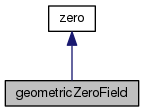[legend]
Collaboration diagram for geometricZeroField:[legend]

## Public Member Functions

geometricZeroField ()
Construct null. More...

const dimensionSetdimensions () const

scalar operator[] (const label) const

zeroField field () const

zeroField oldTime () const

zeroFieldField boundaryField () constPublic Member Functions inherited from zero
zero ()
Construct null. More...

operator bool () const
Return 0 for bool. More...

operator label () const
Return 0 for label. More...

operator float () const
Return 0 for float. More...

operator double () const
Return 0 for double. More...Public Types inherited from zero
typedef zero value_type

## Detailed Description

A class representing the concept of a GeometricField of 1 used to avoid unnecessary manipulations for objects which are known to be zero at compile-time.

Used for example as the density argument to a function written for compressible to be used for incompressible flow.

Definition at line 52 of file geometricZeroField.H.

## Constructor & Destructor Documentation

 geometricZeroField ( )
inline

Construct null.

Definition at line 62 of file geometricZeroField.H.

Here is the call graph for this function: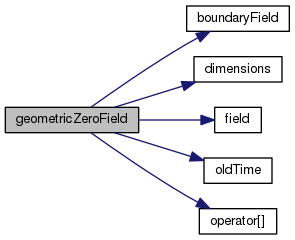## Member Function Documentation

 const Foam::dimensionSet & dimensions ( ) const
inline

Definition at line 30 of file geometricZeroFieldI.H.

References Foam::dimless.

Referenced by geometricZeroField::geometricZeroField().

Here is the caller graph for this function: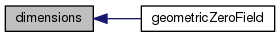Foam::scalar operator[] ( const label ) const
inline

Definition at line 35 of file geometricZeroFieldI.H.

Referenced by geometricZeroField::geometricZeroField().

Here is the caller graph for this function: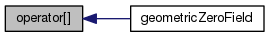Foam::zeroField field ( ) const
inline

Definition at line 40 of file geometricZeroFieldI.H.

Referenced by geometricZeroField::geometricZeroField().

Here is the caller graph for this function: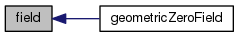Foam::zeroField oldTime ( ) const
inline

Definition at line 45 of file geometricZeroFieldI.H.

Referenced by geometricZeroField::geometricZeroField().

Here is the caller graph for this function: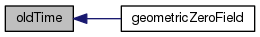Foam::zeroFieldField boundaryField ( ) const
inline

Definition at line 50 of file geometricZeroFieldI.H.

Referenced by geometricZeroField::geometricZeroField().

Here is the caller graph for this function: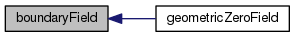The documentation for this class was generated from the following files: